Search IntMath
Close

450+ Math Lessons written by Math Professors and Teachers

5 Million+ Students Helped Each Year

1200+ Articles Written by Math Educators and Enthusiasts

Simplifying and Teaching Math for Over 23 Years

# How to find the equation of a quadratic function from its graph

By Murray Bourne, 17 May 2011

I would like to know how to find the equation of a quadratic function from its graph, including when it does not cut the x-axis. Thanks.

## Modeling

This is a good question because it goes to the heart of a lot of "real" math. Often we have a set of data points from observations in an experiment, say, but we don't know the function that passes through our data points. (Most "text book" math is the wrong way round - it gives you the function first and asks you to plug values into that function.)## A quadratic function's graph is a parabola

The graph of a quadratic function is a parabola. The parabola can either be in "legs up" or "legs down" orientation.

We know that a quadratic equation will be in the form:

y = ax2 + bx + c

Our job is to find the values of a, b and c after first observing the graph. Sometimes it is easy to spot the points where the curve passes through, but often we need to estimate the points.

Let's start with the simplest case. (We'll assume the axis of the given parabola is vertical.)

## Parabola cuts the graph in 2 placesWe can see on the graph that the roots of the quadratic are:

x = −2 (since the graph cuts the x-axis at x = − 2); and

x = 1 (since the graph cuts the x-axis at x = 1.)

Now, we can write our function for the quadratic as follows (since if we solve the following for 0, we'll get our 2 intersection points):

f(x) = (x + 2)(x − 1)

We can expand this to give:

f(x) = x2 + x − 2

This is a quadratic function which passes through the x-axis at the required points.

But is this the correct answer?

Observe my graph passes through −3 on the y-axis. Let's substitute x = 0 into the equation I just got to check if it's correct.

f(0) = 02 + 0 − 2 = −2

It's not correct!

It turns out there are an infinite number of parabolas passing through the points (−2,0) and (1,0).

Here are some of them (in green):And don't forget the parabolas in the "legs down" orientation:So how do we find the correct quadratic function for our original question (the one in blue)?## System of Equations method

To find the unique quadratic function for our blue parabola, we need to use 3 points on the curve. We can then form 3 equations in 3 unknowns and solve them to get the required result.

On the original blue curve, we can see that it passes through the point (0, −3) on the y-axis. We'll use that as our 3rd known point.

Using our general form of the quadratic, y = ax2 + bx + c, we substitute the known values for x and y to obtain:

Substituting (−2,0):

0 = a(−2)2 + b(−2) + c = 4a − 2b + c

Substituting (1,0):

0 = a(1)2 + b(1) + c = a + b + c

Substituting (0,−3):

3 = a(0)2 + b(0) + c = c

So we get c = 3.

Substituting c = 3 in the first line gives:

4a − 2b = 3; and substituting into the second line gives:

a + b = 3

Multiplying the last line by 2 gives:

2a + 2b = 6

Adding this to 4a − 2b = 3 gives:

6a = 9

This gives a = 1.5.

Substituting a = 1.5 into a + b = 3, we get b = 1.5.

So the correct quadratic function for the blue graph is

f(x) = 1.5x2 + 1.5x − 3

We note that the "a" value is positive, resulting in a "legs up" orientation, as expected.

## Vertex method

Another way of going about this is to observe the vertex (the "pointy end") of the parabola.

We can write a parabola in "vertex form" as follows:

y = a(xh)2 + k

For this parabola, the vertex is at (h, k).

In our example above, we can't really tell where the vertex is. It's near (−0.5, −3.4), but "near" will not give us a correct answer. (If there are no other "nice" points where we can see the graph passing through, then we would have to use our estimate.)

The next example shows how we can use the Vertex Method to find our quadratic function.

## One point touching the x-axisThis parabola touches the x-axis at (1, 0) only.

If we use y = a(xh)2 + k, we can see from the graph that h = 1 and k = 0.

This gives us y = a(x − 1)2. What is the value of "a"?

But as in the previous case, we have an infinite number of parabolas passing through (1, 0). Here are some of them:In this example, the blue curve passes through (0, 1) on the y-axis, so we can simply substitute x = 0, y = 1 into y = a(x − 1)2 as follows:

1 = a(− 1)2

This gives us a = 1.

So our quadratic function for this example is

f(x) = (x − 1)2 = x2 − 2x + 1

Note: We could also make use of the fact that the x-value of the vertex of the parabola y = ax2 + bx + c is given by: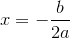## No points touching the x-axis

Here's an example where there is no x-intercept.We can see the vertex is at (-2, 1) and the y-intercept is at (0, 2).

We just substitute as before into the vertex form of our quadratic function.

We have (h, k) = (-2, 1) and at x = 0, y = 2.

So

y = a(xh)2 + k

becomes

2 = a(0 − (−2))2 + 1

2 = 4a +1

a = 0.25

f(x) = 0.25(x −(−2))2 + 1 = 0.25(x + 2)2 + 1 = 0.25(x2 + 4x + 4) + 1

f(x) = 0.25x2 + x + 2

## Using math software to find the function

a. Wolfram|Alpha

This Wolfram|Alpha search gives the answer to my last example.

b. Excel

You could use MS Excel to find the equation. Enter the points in cells as shown, and get Excel to graph it using "X-Y scatter plot". This gives the black curve shown. Then right click on the curve and choose "Add trendline" Choose "Polynomial" and "Order 2". (This gives the blue parabola as shown below).

In the "Options" tab, choose "Display equation on chart".

We get the following result.c. GeoGebra

GeoGebra was not so useful for this task. GeoGebra will give us the equation of a parabola, but you need to know the focus and directrix first. This is not so straightforward from observations of a graph.

## Conclusion

Finding the equation of a parabola given certain data points is a worthwhile skill in mathematics. Parabolas are very useful for mathematical modelling because of their simplicity.### 82 Comments on “How to find the equation of a quadratic function from its graph”

1. Alan Cooper says:

Thanks, once again, for emphasizing "real" math (for both utility and understanding). I agree that this is the kind of thing that schools and texts need to concentrate more on.

Another approach to the parabola problem, which may be of particular interest to calculus students, is that for a parabola to be the graph of y=ax^2+bx+c:
c is the y-intercept (ie the height at the point where x=0)
b is the slope of the tangent line at that point, and
a is the height of the graph above that line at x=1

One of the activities in my "Blue Meanies" game (at http://qpr.ca/math/applets/meanies/ )asks students to "guess" the equation of a parabola through three points by imagining the curve and using its geometry (in various ways) to determine the equation.

2. Murray says:

Thanks for the calculus-based approach, Alan. And thanks for sharing "Meanies"!

3. Henry Bartley says:

I love maths and as a maths student here in DWU university,this lesson send to me is a great help in my learning.

Thank you.

4. alQpr » Blog Archive » How to find the equation of a quadratic function from its graph :: squareCircleZ says:

[...] Bourne of squareCircleZ has posted on ‘How to find the equation of a quadratic function from its graph‘. This is indeed the type of discussion and exercise that we need to see more of.  Not only [...]

5. WITHO JACOB MYEFU says:

Thanks. Its well understood.

6. Lisa A. Gonzales says:

Thanks!!! This helps a lot!!! I agree, as an engineering student this should be a main discussion in all math classes. I am so glad I found this site.

7. Fernando Ireeuw says:

I am a physics and Maths student, and with this lesson sent to me is really a great help in doing quadratics and projectile motion.

Thank you...

8. Math Teachers at Play # 39 « Let's Play Math! says:

[...] Murray Bourne explains step by step How to find the equation of a quadratic function from its graph. [...]

9. Kathryn Peake says:

GeoGebra can be used very easily to find the equation of a parabola: given three points, A, B, C input the command FitPoly[{A, B, C}, 2].

There is also a spreadsheet, which can be used as easily as Excel.

10. Murray says:

Hi Kathryn and thanks for your input. I was not aware of the FitPoly command in GeoGebra - it's a shame it is not included in one of the menus.

11. Mick Stevens says:

I am a 41 year old who is about to study maths and physics at uni for the first time; stuff like this is fantastic.

Keep up the good work.

12. Murray says:

@Mick: Thanks for the positive feedback. Good luck with your studies!

13. Carolyn says:

I am confused about one thing....If the y-intercept is (4.2), would we replace the 4 in place if the x instead of zero....just making sure the 0 is not used every time.

14. Murray says:

@Carolyn: I'm not quite sure what your question means (don't want to lead you astray!).

If we have a y-intercept, the we find it by substituting x = 0. Nothing magic about it - when x does equal zero, we are on the y-axis.

15. John Starkie says:

Very disappointing.
I have no way of calculating x from your final equation without using maths software.
What a cop-out.

16. Murray says:

@John: I don't understand your comment.

This is the final equation in the article: f(x) = 0.25x^2 + x + 2. It is an equation for the parabola shown higher up. Which "x" are you trying to calculate? If you are trying to find the zeros for the function (that is find x when f(x) = 0), then that is simply done using quadratic equation - no need for math software.

The last portion showing how to do it on Wolfram|Alpha, Excel and GeoGebra give us the same answer as on paper. That is, we can do it with software or without. But once again, we are not even trying to find an "x". we are trying to find the equation of the parabola.

17. John Bristow says:

Two questions:
Is there a way to find the formula for a Quartic equation?
Can I use excel and choose polynomial and order 4?

18. Murray says:

@John: Yes, that would do it. The more data points you give Excel (especially near extremes like maxima, minima and x- and y-intercepts), the closer the resulting polynomial will be to your given graph.

19. Jaahnavi Dave says:

Hi, I found your explanation lucid and helpful. I want to know for a set of paired x and y values how do I find vortex points (using f(x)=a(x-h)^2+k formula)? Thanks.

Jaahnavi

20. Murray says:

@Jaahnavi I think you mean "vertex points", right? The vertex occurs where x = h, and that occurs at the lowest (or highest) y-value for your data. Hope it helps!

21. Imani says:

Whoa, this was really helpful! Thanks!!

22. Tarun Songra says:

hi,

Thanks for such a useful information. I am transport planning student and have lot of data where i have to fit parabola. For convenience let us assume that we have 3 points (1,5), (3,2) & (5,3). if we fit these points generally they fit on parabola with axis of symmetry on Y axis but i want to fit these points in parabola with axis of symmetry on X Axis and 2 points of the parabola intersecting on Y Axis.(parabola Legs towards West direction)

More advanced: I want to fit parabola equation at any axis of symmetry.

thanks

23. Murray says:

@Tarun: A very useful tool for you would be GeoGebra.

I did some digging and found a GeoGebra applet (no longer available) which draws a parabola through 3 points. I modified it to give a parabola with horizontal axis through your given 3 points.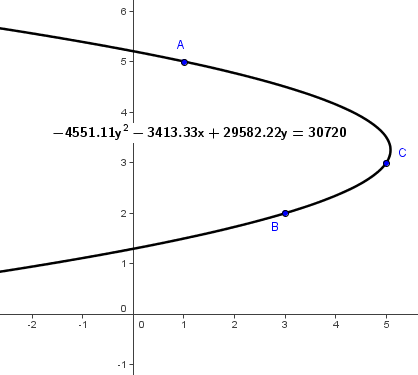You won't be able to fit a parabola with x-axis as the parabola of symmetry through those 3 points - only one parallel to x-axis.

If you are going to try to do it algebraically for many different parabolas it's going to be quite troublesome. GeoGebra is the way to go, I believe. Hope it helps.

24. Abhishek Mishra says:

i have a question where the curve is a parabola passing through the origin a point is given its neither the max nor min it's on the curve the point is (1,2) and then the curve again cuts through the x axis at (6,0)
and i need to form a quadratic equation based on that could you pls help me out with it...........

25. Murray says:

Hello Abhishek. I'm assuming your parabola must have a vertical axis (since you talk about forming a quadratic equation, and this must be in x, since it cannot be in y for your points).

Just go about it the same as I did int he article: start with y = ax^2 + bx + c and substitute in your 3 points, then solve.

Edit: I meant y = ax^2 + bx + c

26. saleem says:

Its really a great job to post about quadratic equation and its curves..i ll recommend it to my colleagues.

27. simon says:

i find just a little problem solving a problem. I am to find a equation of a parablo given the vertex (7,-2) and one x-intercept (4,0). Can you help me with the problem please.

28. Murray says:

@Simon: You'll need to use the "Vertex Method" as detailed in the article. Substitute your known values and you'll end up with a system of equations, similar to the one in the article.

29. Maheera says:

Thanks a lot! I felt sick in Pre-Calc yesterday while they were reviewing this and wasn't up to asking the teacher to repeat everything cuz it didn't make sense at that moment but this really helps ! We already have a quiz Mnday!

30. Murray says:

31. Ethan says:

This really helped; you made me feel better now that I finally know how to do this, so I hope that letting you know you helped another person would make you feel better as well.

32. Murray says:

33. Will says:

I have One question for the first method of systems of equations, where it says "Multiplying the last line by 2 and adding it to the line before gives
a = 1.5 and with that, we easily get b = 1.5."
Which line is the last line that you multiply by 2 and which line do you add that too also how did you get the final answer of 1.5 for A and B? Thanks for all your help

34. Murray says:

@Will: I re-wrote that portion of the solution. I hope it makes more sense now. (You may need to refresh the page to see the revision.)

35. Joe Anderson says:

Although a rather long and drawn out discussion, it might be useful if you offered your readers the method for solving any order polynomial equation using matrix determinants and Cramer's rule. This method will allow one to "fit" a curve to any number of data points. Anything above 4 data points (4x4 matrix) gets really long, but the principle is the same no matter how many data points. I use this method to control the torque profile on a surface driven winder (real world math)
Joe

36. Murray says:

@Joe: See here for Cramer's Rule.

In this day of readily available (and free) computer tools, I no longer recommend Cramer's Rule!

37. Wil says:

Thanks a lot

38. dashk says:

Thanks pal really helped me. May God bless and guide you!
Thanks! Peace!

39. leah says:

how to graph a parabola ? when the only given is the equation??

40. Murray says:

@Leah: These pages will help:

https://www.intmath.com/plane-analytic-geometry/4-parabola.php

41. Marisa says:

I found your graphs and explanations very helpful. But on my math homework, I we are working with conic sections and parabolas. And we have to match the graph to it's corresponding equation. the ones I'm having trouble with are ones like
y^2=-12x or
x^2=2y
How do you solve those kinds?

42. Murray says:

https://www.intmath.com/blog/mathematics/how-to-draw-y2-x-2-2301

43. umer says:

I was about to teach my Masters students about non linear inventory models and came across this simple idea...i must say it is fantastic and now i will teach them this simple technique at the highest level with maximum effecticvity

44. Murray says:

@Umer: I'm glad it was useful!

45. mel says:

Hi! This is super helpful but just wondering, in the systems of equations example, why do multiply the last line by 2? Where are we getting the 2 from and also why would we add it to the second line? Thanks

46. Murray says:

@Mel: It's explained on the line just before that, where it says:

We have (h, k) = (-2, 1) and at x = 0, y = 2.

Those are the values we need to substitute.

47. Peter says:

what if the curve has three x-intecepts? i.e a trinomial?

48. Murray says:

@Peter: Actually, if there are 3 intercepts, it's a quadrinomial.

You would go about it in a similar way. Use the x-intercepts for 3 known values, then choose one other point on the curve and finally, set up a system of 3 equations in 3 unknowns and solve them.

49. Dodi says:

On parabola how can I find the equation of the axis of symmetry .

50. Murray says:

Hello Dodi

https://www.intmath.com/plane-analytic-geometry/4-parabola.php

51. ABC says:

Ive got a question, (sorry for my bad English)

What if there are no points touching the x-axis and y-axis? Like the equation 2(x-3)^2+1? Or maybe it does have but the image provided is limited so I can't see it touching anything and I can't substitute.
How did the value of a become 2? I'm having trouble in determining the equation from its graph (>.<) The image only has 5 units for each positive and negative x and y.

52. Murray says:

@ABC: Every parabola passes through at least one of the axes. In your example, y = 2(x-3)^2+1, when x = 0, y = 19. So the y-intercept is 19.

53. Mike says:

How could we go about figuring out the equation of other types of graphs?

Say I have this quintic polynomial graph without the function. http://www.sscc.edu/home/jdavidso/Math/Catalog/Polynomials/Fifth/FifthDegreeB.html how would I figure out the function?

Or a logarithmic graph, or asymptotic graph if all you have is the graph itself?

54. Murray says:

@Mike: Good question! I'll try to find time to write an article on this.

55. naomi says:

Thank you so much for this page.. Unlike other websites, this one I can actually understand and everything has been so helpful and wonderfully explained 🙂

56. Opal Frances says:

Thank you so, so much for this page! I am in algebra 1 and got stuck on a homework problem. I found this website and it is so wonderful! Unlike most other websites, this is clean, organized, and not overly cluttered with crap. It is neatly listed in order from the top down and was easy to follow. Very well explained, I understood it well after reading it through once. I appreciate the simple images to go along with the explanations, that also helped a lot. Again, thank you so much for putting together this wonderful page for people like me. I will carry this information with me until I forget it, which undoubtably will be very soon, in which case I will soon be back. 🙂

57. thomas says:

May be of interest to some of you, see this simple how-to obtain curve fitting equation from graph image / published graph at:

https://pocketengineer.wordpress.com/2016/04/02/how-to-obtain-curve-fitting-equation-from-graph-image-published-graph/

58. Saranya A says:

Thank you so much Murray Bourne. It was really very helpful.

59. Raka says:

X^2+y^2+axy +bx + cy +k = 0
Does the above equation represent a parabola? What will be the vertex, focus and directrix of such parabola? Please reply soon.

60. Murray says:

Hello Raka. The IntMath Forum would be the appropriate place for your question. Here is the appropriate section: Plane Analytical Geometry. Show your working so we can help you best.

61. haha not my real name says:

My math teacher said to solve for a as much as possible with one section, solve for b as much as possible in another, then uses them to solve eachother by plugging them in to eachother. Everytime i do this i get an infinite loop. Can you help me understand what he means?

62. Murray says:

@haha not my real name: I'm not surprised this ends up in a loop. The best thing is to supply the question and an example given by the teacher so I can see what they mean.

63. Paul says:

In your example where you have the roots as -2 an +1, the factored form you gave was f(x) = (x + 2)(x − 1) and as you noted, this could describe an infinite set of curves

As the y intercept was at -3, could we not simply use this to determine the proper equation: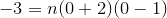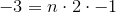Therefore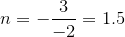Final equation is: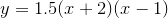Which becomes when expanded: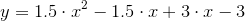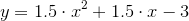64. Suki says:

Hi,
How I can create an Quadratic equation from this?
using the amount of revenues and expenses, we can determine if based on the number of staff and encounters. we are able to determine and establish goals. for instance, we can compare cost per encounter versus revenue by encounters, to find this information we have to total our cost by encounters and compare this to revenue by encounters.

65. Murray says:

@Paul: Yes, that's what I did in the article and arrived at the same equation as you did.

In your example at the top of this page, you end up with the equation (#1), y= x^2+x-2 for the parabola but you rule it out because this equations leads to a y intercept of -2 whereas the graph shows a y intercept of -3. So far, so good. You then go about solving a system of three equations to get the equation(#2): y = 1.5 x^2 + 1.5x - 3.
Instead, you can derive the correct equation (#2) by merely multiplying #1 by 1.5, where 1.5 is the ratio of the correct constant term of -3 to the constant term of -2 in #1.

67. Murray says:

@Madhu: This is the same approach suggested by Paul, a few comments ago.

68. Isabella Stewart says:

System of Equations method;
Hi, I might be seeming a bit stupid here, but how does 6a = 9 = 1.5? I thought you had to divide the 6 by the 9, except that produces a 3.

69. Murray says:

@Isabella. If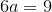, then: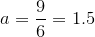I have divided both sides by 6 to give me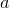on the left. Hope it makes sense.

70. billy says:

f(x) = 0.25(x −(−2))^2 + 1 = 0.25(x + 2)^2 + 1

f(x) = 0.25x2 + x + 2

how do you get 0.25x^2 + x + 2 from 0.25(x + 2)^2 + 1

i don't understand the working, please can you show the steps taken? thank you.

71. Murray says:

@billy: I added an extra line in there for you - hopefully it's clearer now.

72. Harry Dunleavy says:

I am a retired mathematics teacher at H.S and college level with a degree
in mathematics from the City University of New York and a masters from Long Island University. Your work and problems are excellent. By the way, do you know any college that has a doctorate in Mathematics on line as I have nothing else to do. I have tried hard but found none. Again, your page is interesting and excellent for H.S and college students.
Sincerely, Harry Dunleavy

73. Murray says:

I admire your desire to continue learning, however I don't think you'll find a reputable online PhD mathematics program.

I'm wondering whether a role like a research assistant in some existing mathematics education research may be the way to go for you. You would still have the stimulation of collecting and analysing data, without the responsibility of having to write it up at the end (and hopefully you'd get paid...)

Another option could be to approach an existing entity that's doing interesting things (New York's Museum of Mathematics comes to mind) and offer your services as a researcher. They'd probably love it.

But what I'd also hope you'd consider is spending some of your time as a volunteer with students who don't have the means to afford math tutoring, but desperately need it.

Good luck with it.

74. Mathan says:

What if the curve not passing through any of axis. How to find the equation of that curve?

75. Murray says:

@Mathan: What kind of "curve" are you talking about? If you mean it's a parabola, the systems of equations method as given in the post works whether the parabola passes through the x-axis or not. (All parabolas with axis parallel to the y-axis pass through the y-axis).

76. Evogod says:

I don't get this at ALL

77. Murray says:

Hey all,
I am having trouble calculating the function (ax^2 + bx + c) of a parabola. The co-ordinants i have are (-5,0) and (31.26,0) for the x axis, and for the y i have (o,3). The vertex there fore would be (13.13,y?) Then i need to find the function. Can anyone help?

79. Murray says:

@Adam: This would be a good question for the IntMath Forum. Here's the appropriate section: https://www.intmath.com/forum/plane-analytic-geometry-37/

80. Justice says:

Please how can you find the equation of a quadratic curve when given only the plotted values

81. GuQin says:

Do you mind if I quote a few of your articles as long as I provide credit and sources back to your blog? My website is in the very same niche as yours and my users would definitely benefit from some of the information you present here. Please let me know if this ok with you. Thanks a lot!

82. Murray says:

You have permission to link to IntMath, but you cannot copy articles to your own site. I have spent many years developing the materials in IntMath - please respect that work.

### Comment Preview

HTML: You can use simple tags like <b>, <a href="...">, etc.

To enter math, you can can either:

1. Use simple calculator-like input in the following format (surround your math in backticks, or qq on tablet or phone):
a^2 = sqrt(b^2 + c^2)
(See more on ASCIIMath syntax); or
2. Use simple LaTeX in the following format. Surround your math with $$ and $$.
$$\int g dx = \sqrt{\frac{a}{b}}$$
(This is standard simple LaTeX.)

NOTE: You can mix both types of math entry in your comment.